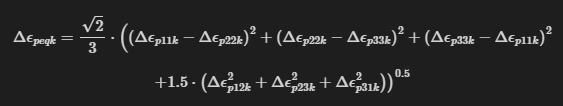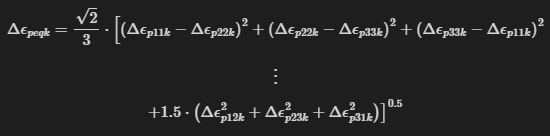# Add newline to LaTeXString equation

Hello, I have a LaTeXString which is too long to fit in one page-width. How can I add a line break to the equation so that it fits? I tried to add a \\ in the middle, but that didn’t change the rendering at all.

julia> s = L"$\Delta\epsilon_{peqk} = \frac{\sqrt{2}}{3} \cdot \left( \left( \Delta\epsilon_{p11k} - \Delta\epsilon_{p22k} \right)^{2} + \left( \Delta\epsilon_{p22k} - \Delta\epsilon_{p33k} \right)^{2} + \left( \Delta\epsilon_{p33k} - \Delta\epsilon_{p11k} \right)^{2} + 1.5 \cdot \left( \Delta\epsilon_{p12k}^{2} + \Delta\epsilon_{p23k}^{2} + \Delta\epsilon_{p31k}^{2} \right) \right)^{0.5}$";

julia> ss = LaTeXString(replace(s, "+ 1.5" => "\\\\ + 1.5"; count=1))
L"$\Delta\epsilon_{peqk} = \frac{\sqrt{2}}{3} \cdot \left( \left( \Delta\epsilon_{p11k} - \Delta\epsilon_{p22k} \right)^{2} + \left( \Delta\epsilon_{p22k} - \Delta\epsilon_{p33k} \right)^{2} + \left( \Delta\epsilon_{p33k} - \Delta\epsilon_{p11k} \right)^{2} \\ + 1.5 \cdot \left( \Delta\epsilon_{p12k}^{2} + \Delta\epsilon_{p23k}^{2} + \Delta\epsilon_{p31k}^{2} \right) \right)^{0.5}$"

julia> render(ss)


Rendered in VSCode:

Rendered to PDF with Weave:

I’m not sure that even works in plain Latex. Typically you need to use something a bit fancier like align or multiline, right?

Probably, but I’m not sure how to do something that fancy with Latexify and LaTeXStrings. Anything that keeps it on the page would be good enough for me.

This is kind of working, but I still think I am going to lose some to the margin if I print. (I could not split where I originally wanted to because it will not let me leave an open parenthesis.)

(s1, s2) = split(s, "= ")
s1 = LaTeXString(s1 * "=\$") s2 = LaTeXString("\$" * s2)
render(s1)
render(s2)


There is a related closed Issue for Weave here, but I don’t understand how to make it work.

Personally, I would not spend too much time on this. May I suggest that you break your equation into several? Like:

A =B+C+D+...
where
B = ...
C = ...
and D= ...

It is often easier to read.
It the case whare each term has an interpretation, then it is evan better becaus you can comment on B, C or D without resorting to ugly contortions like “the second term of the righthand side”.

Of course you don’t get all in one string but you get to decide where you render them.

Just a thought.

1 Like

Not sure where you got to on this. Does this work for you?

using LaTeXStrings
import Latexify

s = L"""
\Delta\epsilon_{peqk} = \frac{\sqrt{2}}{3} \cdot \left( \left( \Delta\epsilon_{p11k} - \Delta\epsilon_{p22k} \right)^{2} + \left( \Delta\epsilon_{p22k} - \Delta\epsilon_{p33k} \right)^{2} + \left( \Delta\epsilon_{p33k} - \Delta\epsilon_{p11k} \right)^{2} \right. \\
+ \left. 1.5 \cdot \left( \Delta\epsilon_{p12k}^{2} + \Delta\epsilon_{p23k}^{2} + \Delta\epsilon_{p31k}^{2} \right) \right)^{0.5}
"""

Latexify.render(s)


Note the balancing of \left( with \right. and vice versa on the second.

1 Like

Copying your code directly, the equation still appears on a single line as before.
However, if I add $ around each line and delete \\, it works pretty well. Thanks. s = L"""$\Delta\epsilon_{peqk} = \frac{\sqrt{2}}{3} \cdot \left( \left( \Delta\epsilon_{p11k} - \Delta\epsilon_{p22k} \right)^{2} + \left( \Delta\epsilon_{p22k} - \Delta\epsilon_{p33k} \right)^{2} + \left( \Delta\epsilon_{p33k} - \Delta\epsilon_{p11k} \right)^{2} \right.+ \left. 1.5 \cdot \left( \Delta\epsilon_{p12k}^{2} + \Delta\epsilon_{p23k}^{2} + \Delta\epsilon_{p31k}^{2} \right) \right)^{0.5}$"""Note that that has nothing to do with LaTexStrings per se, and everything to do with whatever is doing the LaTeX rendering in your environment (e.g. MathJax in Jupyter). The downside to this approach is that the last \right) does not realize it should be the same size as the first \left(, but I am tempted to call this good enough. s = L"""$\Delta\epsilon_{peqk} = \frac{\sqrt{2}}{3} \cdot \left[ \left( \Delta\epsilon_{p11k} - \Delta\epsilon_{p22k} \right)^{2} + \left( \Delta\epsilon_{p22k} - \Delta\epsilon_{p33k} \right)^{2} + \left( \Delta\epsilon_{p33k} - \Delta\epsilon_{p11k} \right)^{2} \right.\vdots+ \left. 1.5 \cdot \left( \Delta\epsilon_{p12k}^{2} + \Delta\epsilon_{p23k}^{2} + \Delta\epsilon_{p31k}^{2} \right) \right]^{0.5}$""" render(s)1 Like I had the same problem in the title of a plot. I solved by concatenating the LaTeXStrings with a regular string that contains a newline at the appropiate place: t = L"Displacement$\delta(\theta)$"*"\n"*L"and rotation$\omega_\varphi(\theta)$" plot([sin cos],pi/2,0.999*pi,title=t, label=[L"$\delta(\theta)$" L"$\omega_\varphi\$"], xlabel = "θ (rad)")

2 Likes